# 4.1 Linear functions  (Page 16/27)

 Page 16 / 27

Write an equation for a line perpendicular to $\text{\hspace{0.17em}}h\left(t\right)=-2t+4\text{\hspace{0.17em}}$ and passing through the point $\text{\hspace{0.17em}}\left(-4,–1\right).$

Write an equation for a line perpendicular to $\text{\hspace{0.17em}}p\left(t\right)=3t+4\text{\hspace{0.17em}}$ and passing through the point $\text{\hspace{0.17em}}\left(3,1\right).$

$y=-\frac{1}{3}t+2$

## Graphical

For the following exercises, find the slope of the line graphed.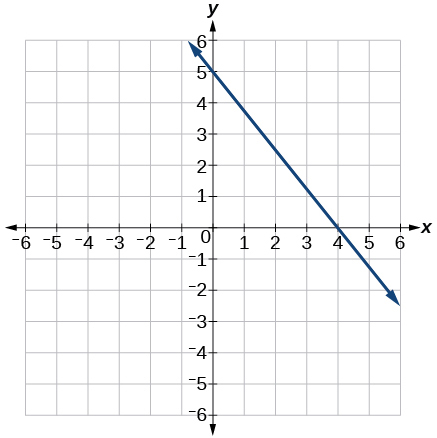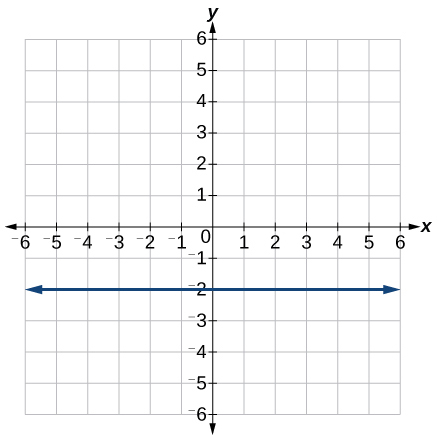0

For the following exercises, write an equation for the line graphed.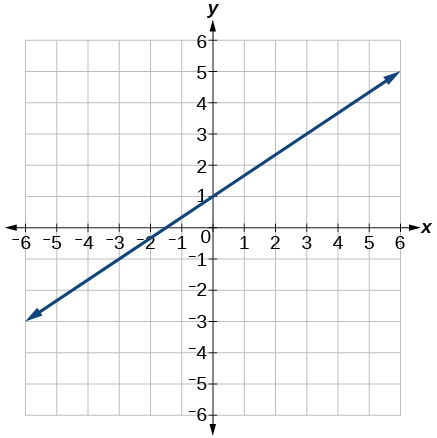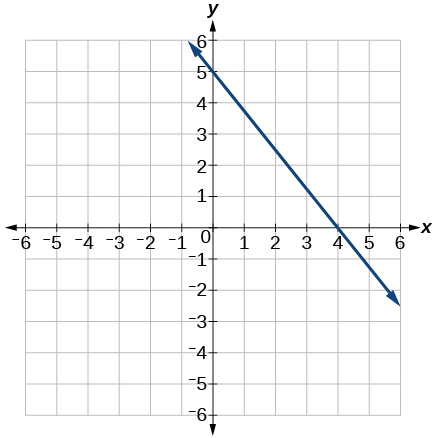$y=-\frac{5}{4}x+5$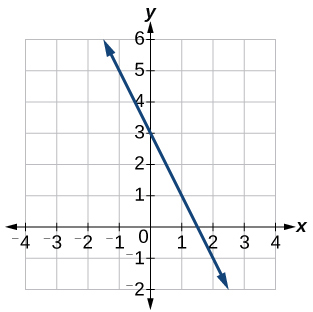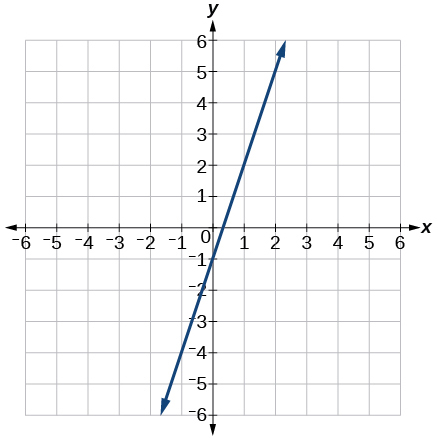$y=3x-1$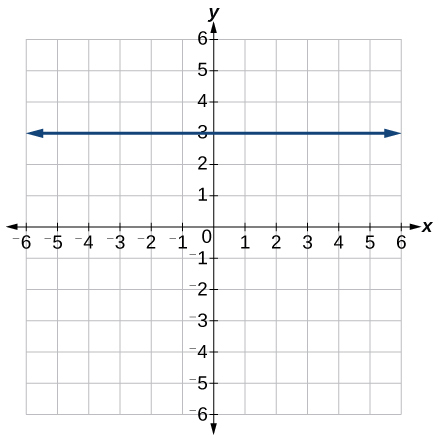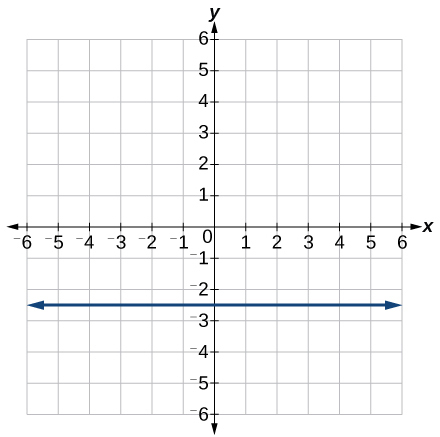$y=-2.5$

For the following exercises, match the given linear equation with its graph in [link] .

$f\left(x\right)=-x-1$

$f\left(x\right)=-2x-1$

F

$f\left(x\right)=-\frac{1}{2}x-1$

$f\left(x\right)=2$

C

$f\left(x\right)=2+x$

$f\left(x\right)=3x+2$

A

For the following exercises, sketch a line with the given features.

An x -intercept of $\text{\hspace{0.17em}}\left(–4,\text{0}\right)\text{\hspace{0.17em}}$ and y -intercept of $\text{\hspace{0.17em}}\left(0,\text{–2}\right)$

An x -intercept $\text{\hspace{0.17em}}\left(–2,\text{0}\right)\text{\hspace{0.17em}}$ and y -intercept of $\text{\hspace{0.17em}}\left(0,\text{4}\right)$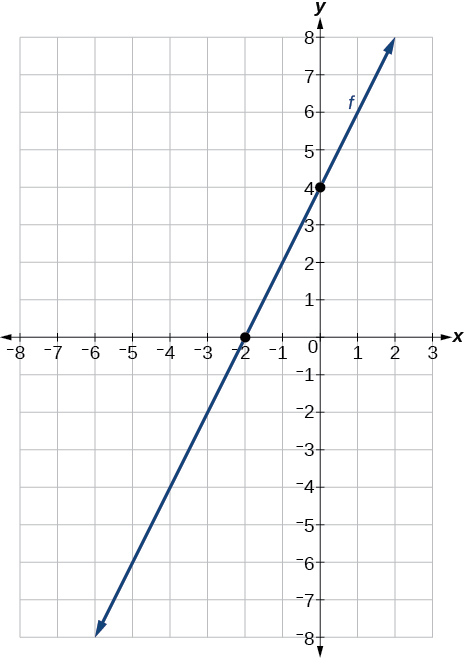A y -intercept of $\text{\hspace{0.17em}}\left(0,\text{7}\right)\text{\hspace{0.17em}}$ and slope $\text{\hspace{0.17em}}-\frac{3}{2}$

A y -intercept of $\text{\hspace{0.17em}}\left(0,\text{3}\right)\text{\hspace{0.17em}}$ and slope $\text{\hspace{0.17em}}\frac{2}{5}$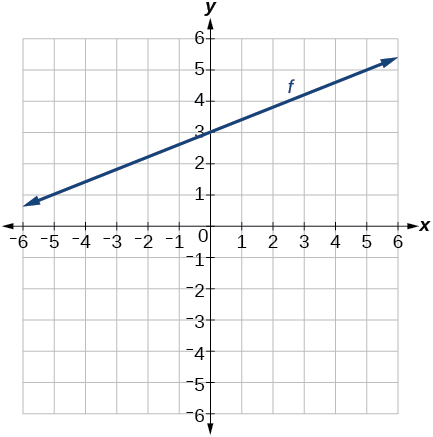Passing through the points $\text{\hspace{0.17em}}\left(–6,\text{–2}\right)\text{\hspace{0.17em}}$ and $\text{\hspace{0.17em}}\left(6,\text{–6}\right)$

Passing through the points $\text{\hspace{0.17em}}\left(–3,\text{–4}\right)\text{\hspace{0.17em}}$ and $\text{\hspace{0.17em}}\left(3,\text{0}\right)$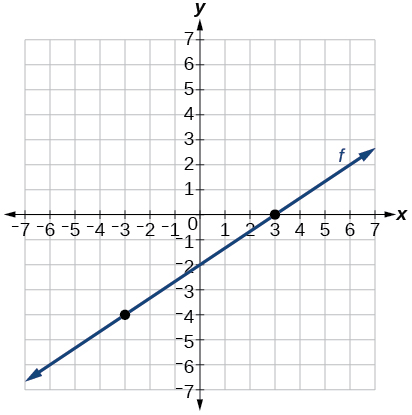For the following exercises, sketch the graph of each equation.

$f\left(x\right)=-2x-1$

$f\left(x\right)=-3x+2$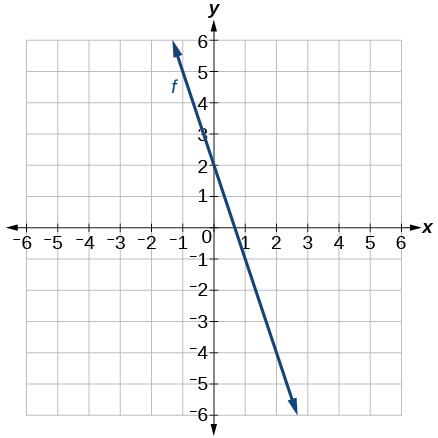$f\left(x\right)=\frac{1}{3}x+2$

$f\left(x\right)=\frac{2}{3}x-3$$f\left(t\right)=3+2t$

$p\left(t\right)=-2+3t$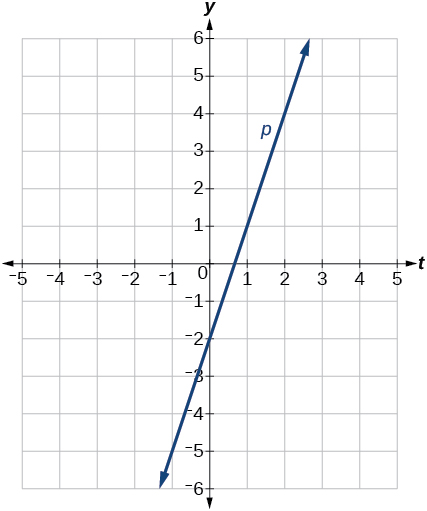$x=3$

$x=-2$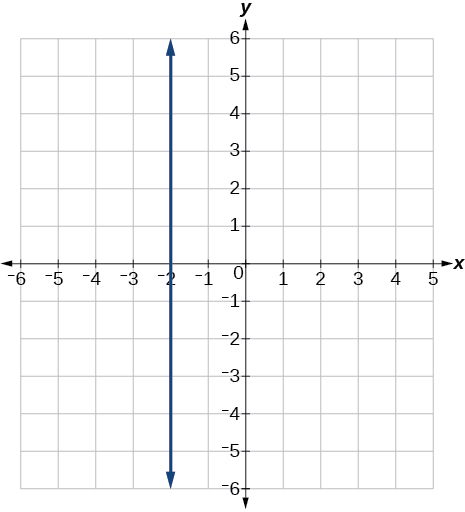$r\left(x\right)=4$

For the following exercises, write the equation of the line shown in the graph.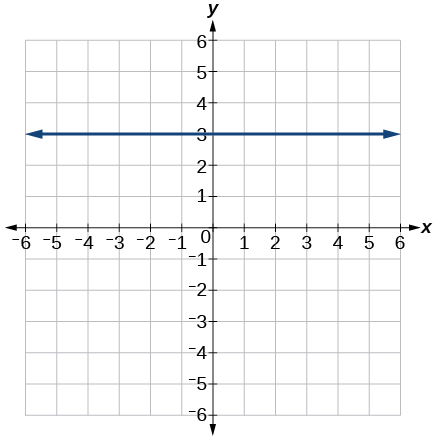$y=\text{3}$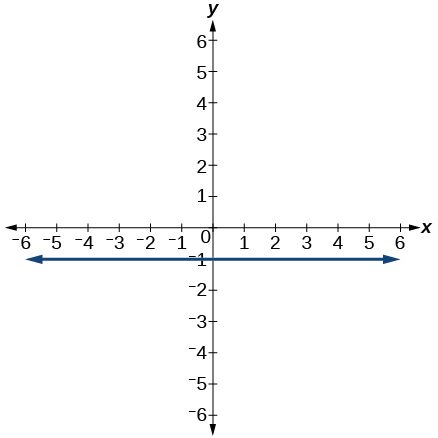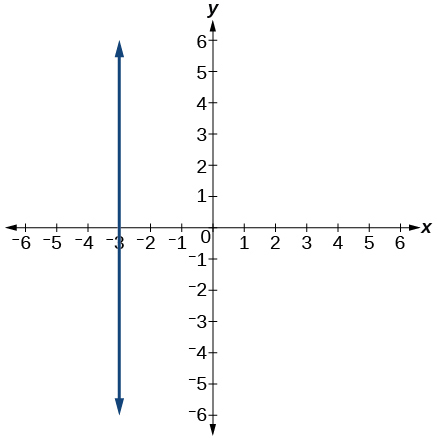$x=-3$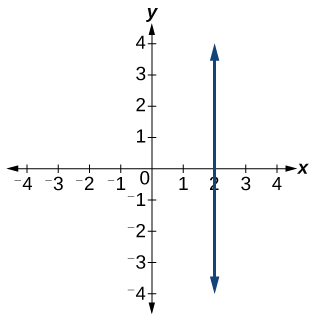## Numeric

For the following exercises, which of the tables could represent a linear function? For each that could be linear, find a linear equation that models the data.

 $x$ 0 5 10 15 $g\left(x\right)$ 5 –10 –25 –40

Linear, $\text{\hspace{0.17em}}g\left(x\right)=-3x+5$

 $x$ 0 5 10 15 $h\left(x\right)$ 5 30 105 230
 $x$ 0 5 10 15 $f\left(x\right)$ –5 20 45 70

Linear, $\text{\hspace{0.17em}}f\left(x\right)=5x-5$

 $x$ 5 10 20 25 $k\left(x\right)$ 13 28 58 73
 $x$ 0 2 4 6 $g\left(x\right)$ 6 –19 –44 –69

Linear, $\text{\hspace{0.17em}}g\left(x\right)=-\frac{25}{2}x+6$

 $x$ 2 4 8 10 $h\left(x\right)$ 13 23 43 53
 $x$ 2 4 6 8 $f\left(x\right)$ –4 16 36 56

Linear, $\text{\hspace{0.17em}}f\left(x\right)=10x-24$

 $x$ 0 2 6 8 $k\left(x\right)$ 6 31 106 231

## Technology

For the following exercises, use a calculator or graphing technology to complete the task.

If $\text{\hspace{0.17em}}f\text{\hspace{0.17em}}$ is a linear function, find an equation for the function.

$f\left(x\right)=-58x+17.3$

Graph the function $\text{\hspace{0.17em}}f\text{\hspace{0.17em}}$ on a domain of $\text{\hspace{0.17em}}\left[–10,10\right]:f\left(x\right)=0.02x-0.01.\text{\hspace{0.17em}}$ Enter the function in a graphing utility. For the viewing window, set the minimum value of $\text{\hspace{0.17em}}x\text{\hspace{0.17em}}$ to be $\text{\hspace{0.17em}}-10\text{\hspace{0.17em}}$ and the maximum value of $\text{\hspace{0.17em}}x\text{\hspace{0.17em}}$ to be $\text{\hspace{0.17em}}10.$

Graph the function $\text{\hspace{0.17em}}f\text{\hspace{0.17em}}$ on a domain of $\text{\hspace{0.17em}}\left[–10,10\right]:fx\right)=2,500x+4,000$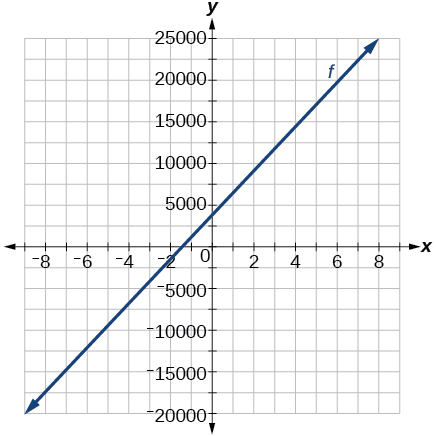[link] shows the input, $\text{\hspace{0.17em}}w,$ and output, $\text{\hspace{0.17em}}k,$ for a linear function $\text{\hspace{0.17em}}k.\text{\hspace{0.17em}}$ a. Fill in the missing values of the table. b. Write the linear function $\text{\hspace{0.17em}}k,$ round to 3 decimal places.

 w –10 5.5 67.5 b k 30 –26 a –44

[link] shows the input, $\text{\hspace{0.17em}}p,$ and output, $\text{\hspace{0.17em}}q,$ for a linear function $\text{\hspace{0.17em}}q.\text{\hspace{0.17em}}$ a. Fill in the missing values of the table. b. Write the linear function $\text{\hspace{0.17em}}k.$

 p 0.5 0.8 12 b q 400 700 a 1,000,000

Graph the linear function $\text{\hspace{0.17em}}f\text{\hspace{0.17em}}$ on a domain of $\text{\hspace{0.17em}}\left[-10,10\right]\text{\hspace{0.17em}}$ for the function whose slope is $\text{\hspace{0.17em}}\frac{1}{8}\text{\hspace{0.17em}}$ and y -intercept is $\text{\hspace{0.17em}}\frac{31}{16}.\text{\hspace{0.17em}}$ Label the points for the input values of $\text{\hspace{0.17em}}-10\text{\hspace{0.17em}}$ and $\text{\hspace{0.17em}}10.$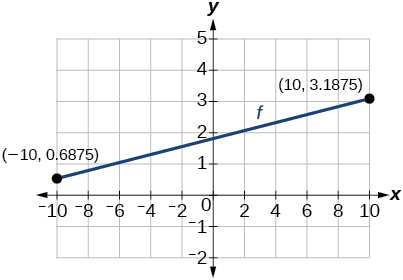Graph the linear function $\text{\hspace{0.17em}}f\text{\hspace{0.17em}}$ on a domain of $\text{\hspace{0.17em}}\left[-0.1,0.1\right]\text{\hspace{0.17em}}$ for the function whose slope is 75 and y -intercept is $\text{\hspace{0.17em}}-22.5.\text{\hspace{0.17em}}$ Label the points for the input values of $\text{\hspace{0.17em}}-0.1\text{\hspace{0.17em}}$ and $\text{\hspace{0.17em}}0.1.$

Graph the linear function $\text{\hspace{0.17em}}f\text{\hspace{0.17em}}$ where $\text{\hspace{0.17em}}f\left(x\right)=ax+b\text{\hspace{0.17em}}$ on the same set of axes on a domain of $\text{\hspace{0.17em}}\left[-4,4\right]\text{\hspace{0.17em}}$ for the following values of $\text{\hspace{0.17em}}a\text{\hspace{0.17em}}$ and $\text{\hspace{0.17em}}b.$

1. $a=2;b=3$
2. $a=2;b=4$
3. $a=2;b=–4$
4. $a=2;b=–5$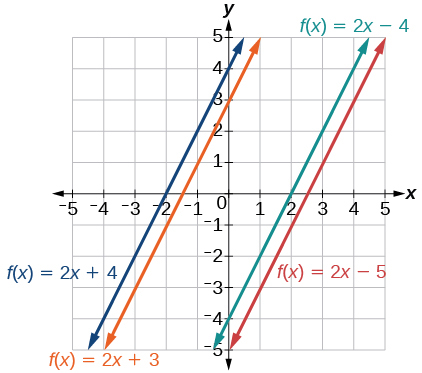## Extensions

Find the value of $\text{\hspace{0.17em}}x\text{\hspace{0.17em}}$ if a linear function goes through the following points and has the following slope: $\text{\hspace{0.17em}}\left(x,2\right),\left(-4,6\right),\text{\hspace{0.17em}}m=3$

Find the value of y if a linear function goes through the following points and has the following slope: $\text{\hspace{0.17em}}\left(10,y\right),\left(25,100\right),\text{\hspace{0.17em}}m=-5$

y = 175

#### Questions & Answers

if sin15°=√p, express the following in terms of p
Norman Reply
prove sin²x+cos²x=3+cos4x
Kiddy Reply
the difference between two signed numbers is -8.if the minued is 5,what is the subtrahend
jeramie Reply
the difference between two signed numbers is -8.if the minuend is 5.what is the subtrahend
jeramie
what are odd numbers
micheal Reply
numbers that leave a remainder when divided by 2
Thorben
1,3,5,7,... 99,...867
Thorben
7%2=1, 679%2=1, 866245%2=1
Thorben
the third and the seventh terms of a G.P are 81 and 16, find the first and fifth terms.
Suleiman Reply
if a=3, b =4 and c=5 find the six trigonometric value sin
Martin Reply
ask
Ans
pls how do I factorize x⁴+x³-7x²-x+6=0
Gift Reply
in a function the input value is called
Rimsha Reply
how do I test for values on the number line
Modesta Reply
if a=4 b=4 then a+b=
Rimsha Reply
a+b+2ab
Kin
commulative principle
DIOSDADO
a+b= 4+4=8
Mimi
If a=4 and b=4 then we add the value of a and b i.e a+b=4+4=8.
Tariq
what are examples of natural number
sani Reply
an equation for the line that goes through the point (-1,12) and has a slope of 2,3
Katheryn Reply
3y=-9x+25
Ishaq
show that the set of natural numberdoes not from agroup with addition or multiplication butit forms aseni group with respect toaaddition as well as multiplication
Komal Reply
x^20+x^15+x^10+x^5/x^2+1
Urmila Reply
evaluate each algebraic expression. 2x+×_2 if ×=5
Sarch Reply

### Read also:

#### Get Jobilize Job Search Mobile App in your pocket Now!

Source:  OpenStax, Algebra and trigonometry. OpenStax CNX. Nov 14, 2016 Download for free at https://legacy.cnx.org/content/col11758/1.6
Google Play and the Google Play logo are trademarks of Google Inc.

Notification Switch

Would you like to follow the 'Algebra and trigonometry' conversation and receive update notifications?ByByBy Anh DaoBy OpenStaxBy Eric CrawfordBy Rohini AjayBy John GabrieliBy OpenStaxBy OpenStaxBy John GabrieliByBy Inderjeet Brar